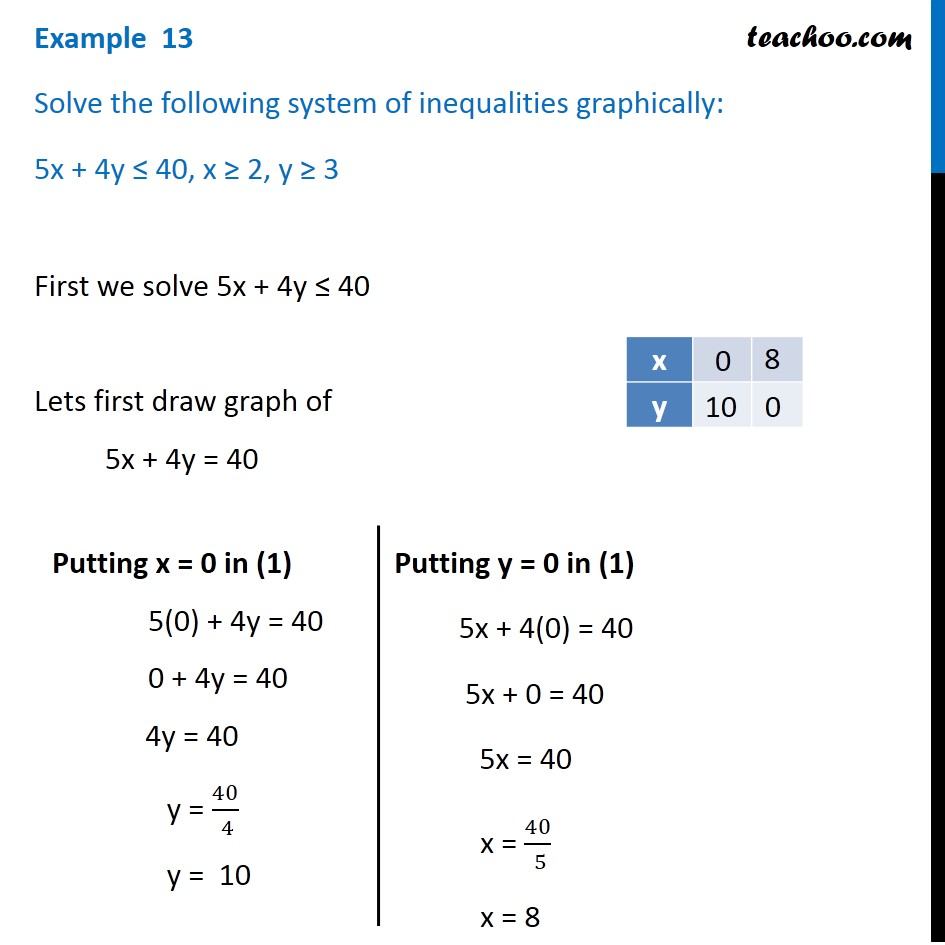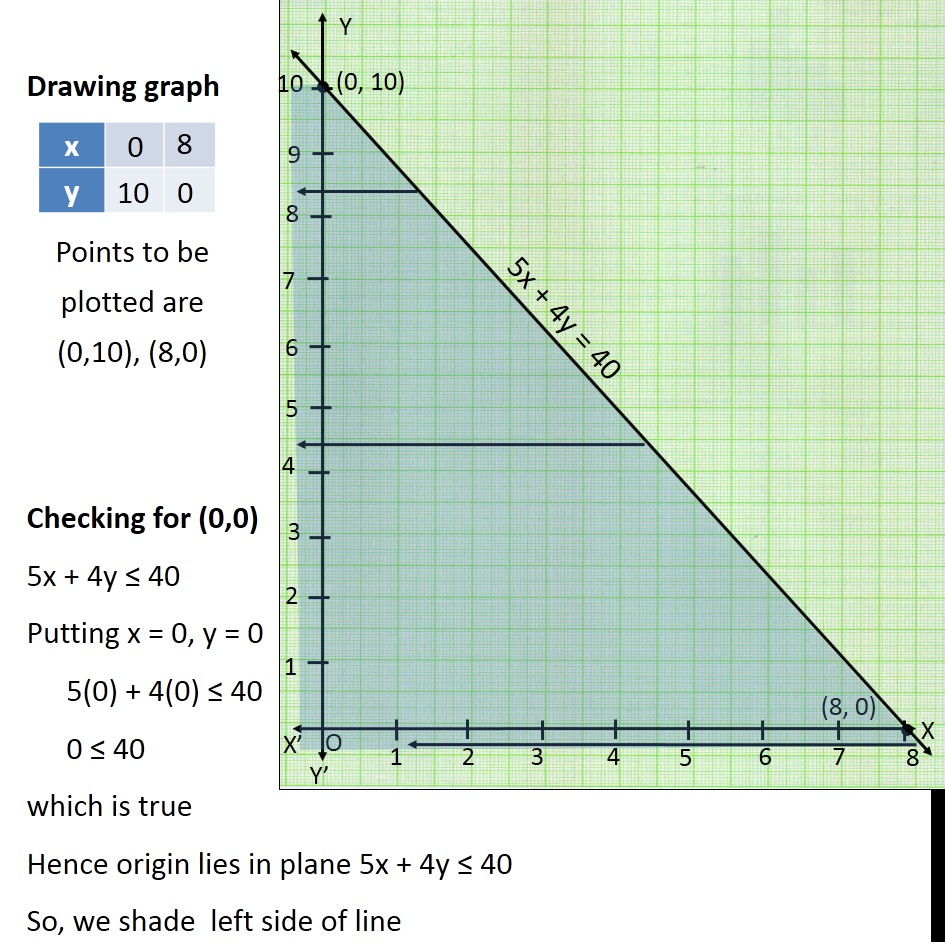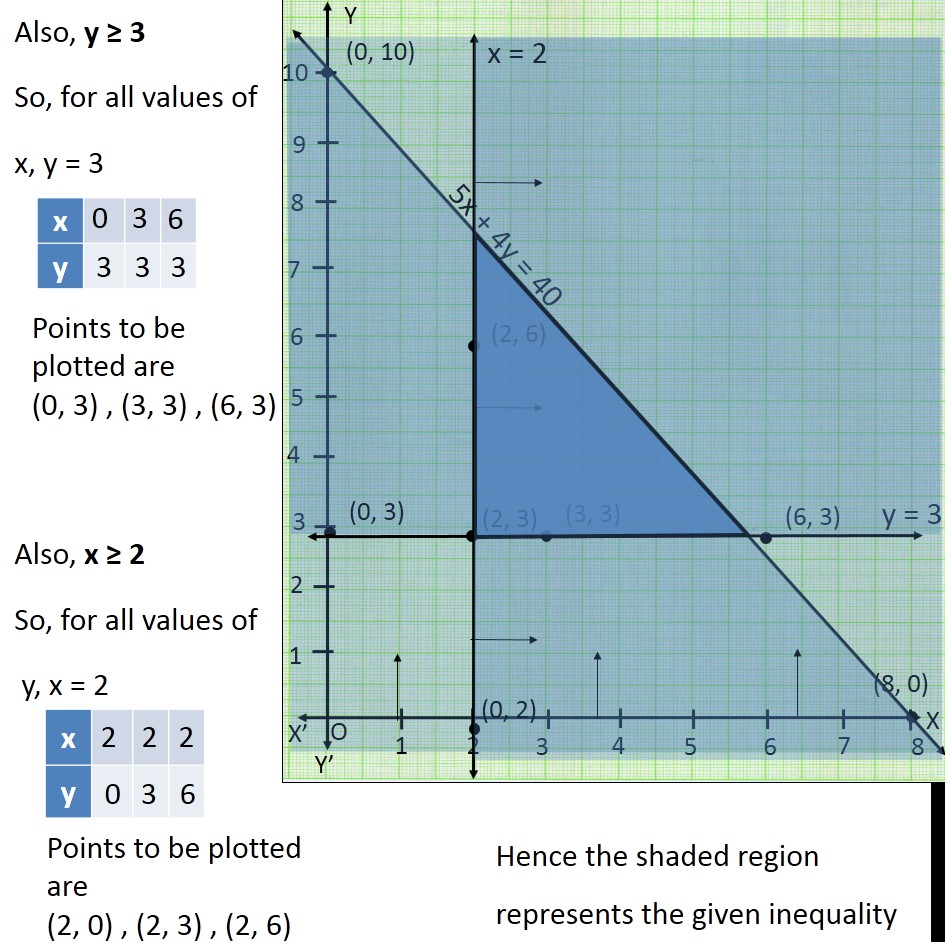Examples

Chapter 5 Class 11 Linear Inequalities
Serial order wiseLearn in your speed, with individual attention - Teachoo Maths 1-on-1 Class

### Transcript

Question 5 Solve the following system of inequalities graphically: 5x + 4y ≤ 40, x ≥ 2, y ≥ 3 First we solve 5x + 4y ≤ 40 Lets first draw graph of 5x + 4y = 40 Putting x = 0 in (1) 5(0) + 4y = 40 0 + 4y = 40 4y = 40 y = 40/( 4) y = 10 Putting y = 0 in (1) 5x + 4(0) = 40 5x + 0 = 40 5x = 40 x = 40/( 5) x = 8 Points to be plotted are (0,10), (8,0) Drawing graph Checking for (0,0) 5x + 4y ≤ 40 Putting x = 0, y = 0 5(0) + 4(0) ≤ 40 0 ≤ 40 which is true Hence origin lies in plane 5x + 4y ≤ 40 So, we shade left side of line Points to be plotted are (0, 3) , (3, 3) , (6, 3) Points to be plotted are (0, 3) , (3, 3) , (6, 3) Points to be plotted are (2, 0) , (2, 3) , (2, 6) Hence the shaded region represents the given inequality